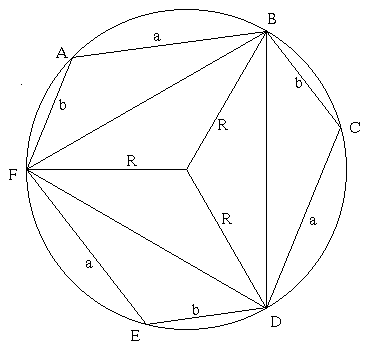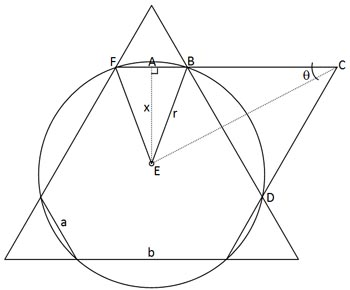#### You may also likeFour rods are hinged at their ends to form a convex quadrilateral. Investigate the different shapes that the quadrilateral can take. Be patient this problem may be slow to load.Given a square ABCD of sides 10 cm, and using the corners as centres, construct four quadrants with radius 10 cm each inside the square. The four arcs intersect at P, Q, R and S. Find the area enclosed by PQRS.### Get Cross

A white cross is placed symmetrically in a red disc with the central square of side length sqrt 2 and the arms of the cross of length 1 unit. What is the area of the disc still showing?

# Hexy-metry

##### Age 14 to 16 Challenge Level:

This very good solution came from The Maths Club at Wilson's School :

The interior angles of the hexagon are all equal to 120 degrees by symmetry.

Let O be the centre of the circle, with radius $r$. Considering triangle BCD, by the cosine rule,
$$BD^2 = a^2 + b^2 - 2ab \cos 120.$$
Considering triangle BOD, by the cosine rule,
$$BD^2 = 2r^2 - 2r^2 \cos 120.$$

As $\cos 120 = -\frac {1}{2}$, we have $a^2 + b^2 + ab = 3r^2$ so
$$r = \sqrt {{a^2 + b^2 + ab\over 3}}.$$Ruth from the Manchester High School for Girls proves the figure has rotational symmetry of order 3 and the angles are 120 degrees as follows, and then goes on to find the solution using the Cosine Rule as above: Let O be the centre of the circle and $R$ be the radius. Construct the radii OA, OB, OC, OD, OE and OF. This creates 6 isoceles triangles, 3 with sides $R, R$ and $a$ and 3 with sides $R, R$ and $b$, therefore by SSS congruence, angles FAO=AFO=CBO=BCO=DEO=EDO and BAO=ABO=CDO=DCO=FEO=EFO therefore the angles of the hexagon are all 120 degrees. ACE and FBD are therfore equilateral triangles.
 Matt, who did not reveal his school, used a different method not involving the Cosine Rule. This hexagon is formed by drawing an equilateral triangle over a circle where the centre of the circle coincides with the centre of the triangle and joining the points at which the triangle crosses the circle. The shorter edge of the hexagon has length $FB= a$ and the longer has length $b$. Therefore line AB has length $\frac {a}{2}$ and line BD has length $b$. Constructing the equilateral triangle BCD defines line BC as having length $b$. So line AC has length $\frac{a}{2}+b$.Let line AE have length $x$. The radius has length $r$. From triangle ABE and using Pythagoras:
$$r^2 = x^2 +\left({\frac{a}{2}}\right)^2.$$
From triangle ACE: $$\tan \theta = \frac{x}{\frac{a}{2} +b}$$ but, $\theta = 30^o$ (since it is half the internal angle of an equilateral triangle) so, $$x = \frac{1}{\sqrt 3}\left( \frac{a}{2} +b \right).$$ Therefore, by eliminating $x$ between these expressions and simplifying, we get:
$$r = \sqrt {{a^2 + b^2 + ab\over 3}}.$$